Next: Elastic response theory Up: Yielding of an elastic Previous: Surface harmonics and solid

# Gravitational potential theory

Consider a spherical planet whose unperturbed surface corresponds to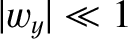. Suppose that this planet is subject to a small, externally generated, tidal potential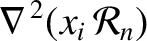, where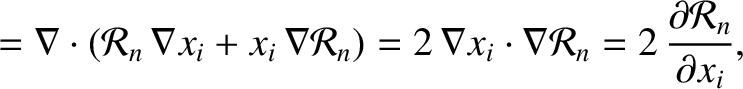is a solid harmonic of degree 2. The planet is modeled as a solid body of uniform mass density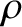whose perturbed surface lies at(C.8)

where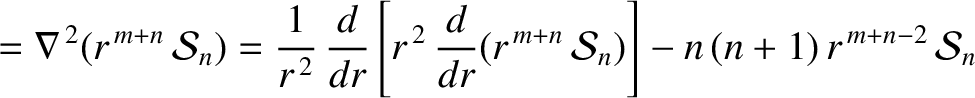is a surface harmonic of degree 2. It is assumed that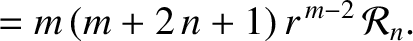.

The net gravitational acceleration in the vicinity of the planet takes the form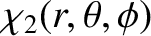(C.9)

where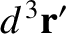is the gravitational potential. According to standard gravitational theory,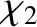(C.10)

where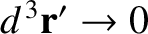is the mass density distribution. Thus, we can write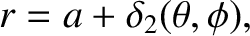(C.11)

where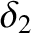(C.12)

and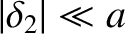(C.13)

[See Equations (E.2) and (E.3).] Here,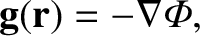is a Dirac delta function (Riley 1974b). The physical boundary conditions are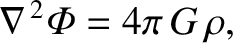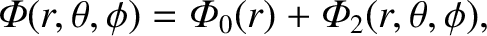(C.14) and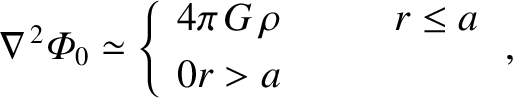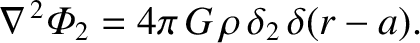(C.15)

as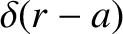. As is well known, the solutions to Laplace's equation,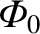, take the general form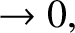and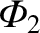. Moreover, the axisymmetric solution to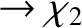that is well behaved at the origin is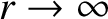. (Riley 1974c.) It follows that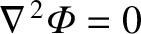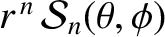(C.16)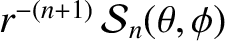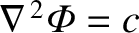(C.17)

and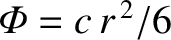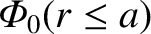(C.18)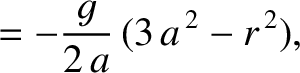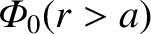(C.19)

where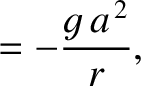(C.20)

is the gravitational acceleration at the planet's surface. Note that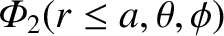is a solid harmonic of degree 2 inside the planet (i.e.,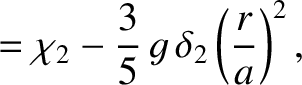).Next: Elastic response theory Up: Yielding of an elastic Previous: Surface harmonics and solid
Richard Fitzpatrick 2016-03-31